Examples

Chapter 10 Class 11 Straight Lines
Serial order wise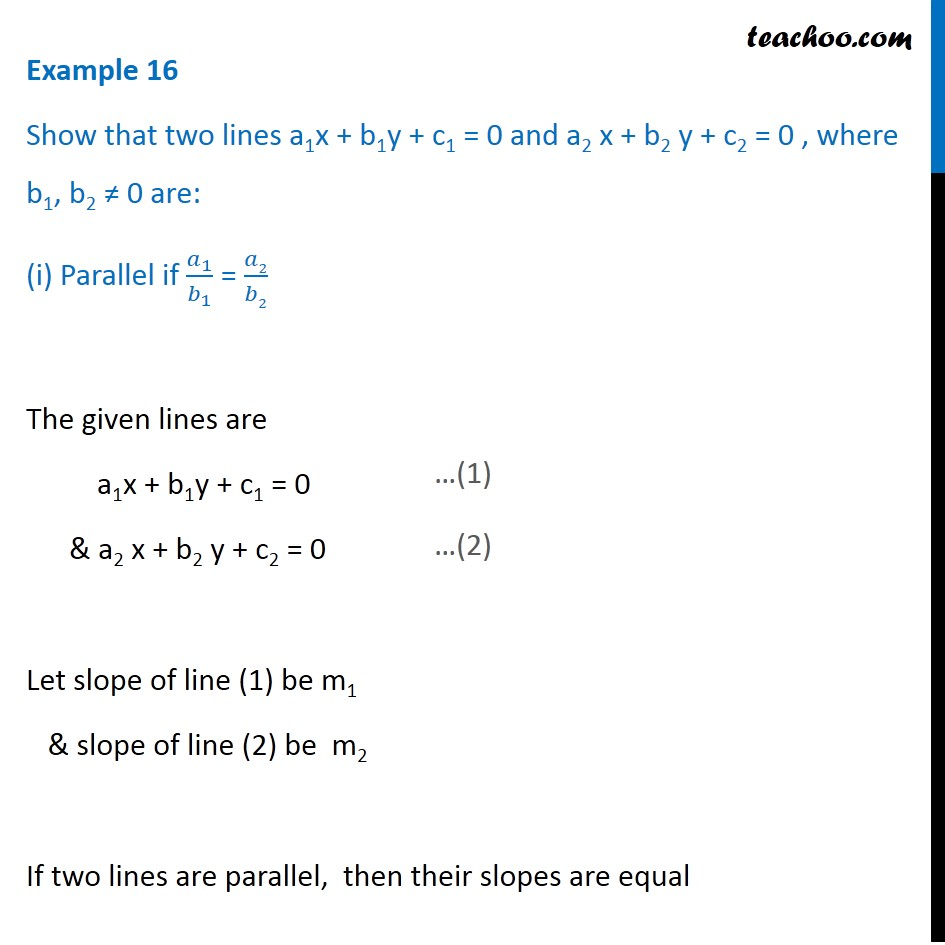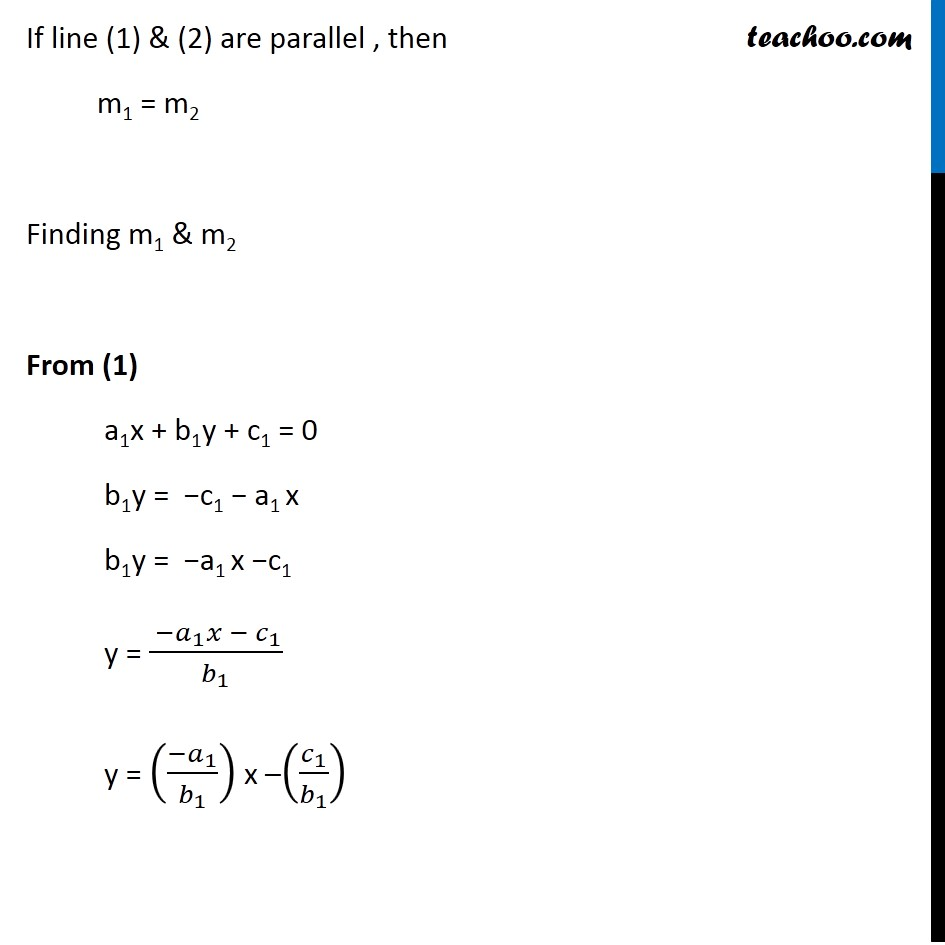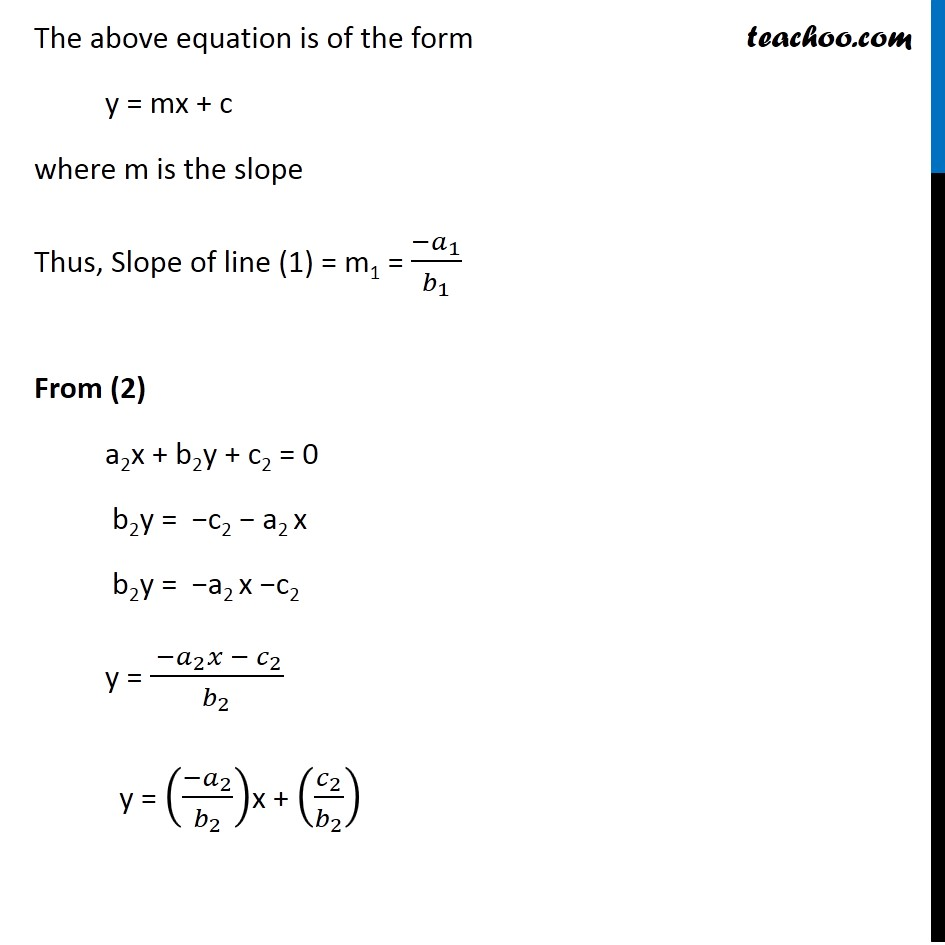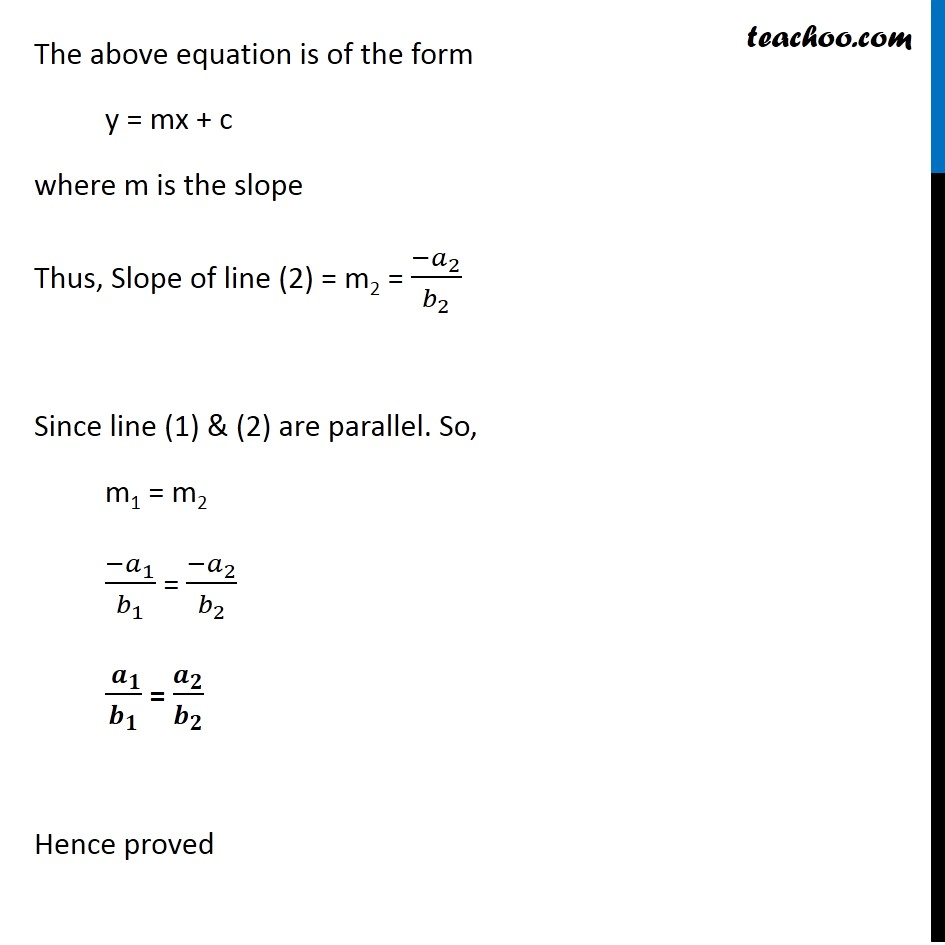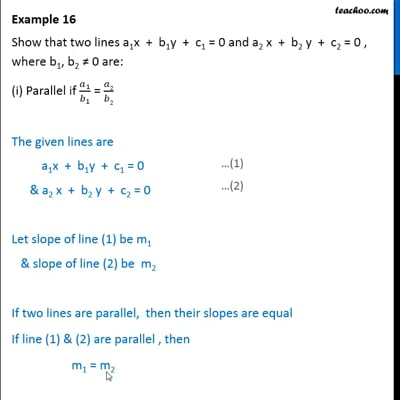This video is only available for Teachoo black users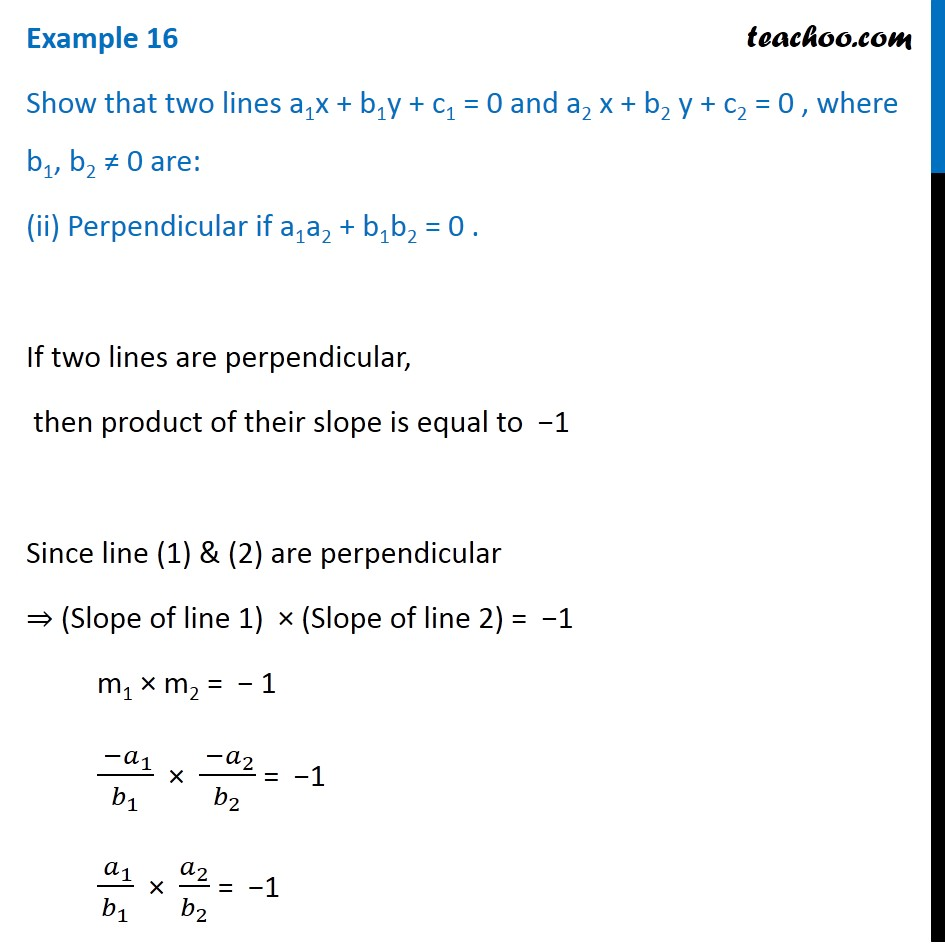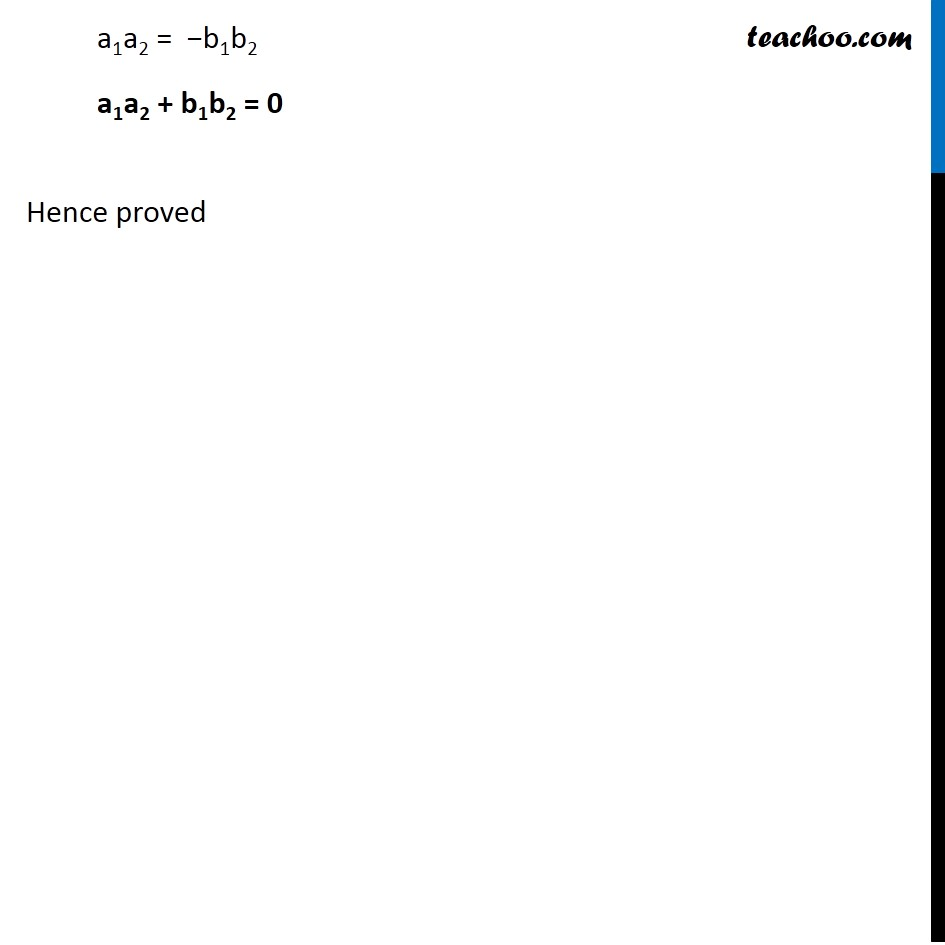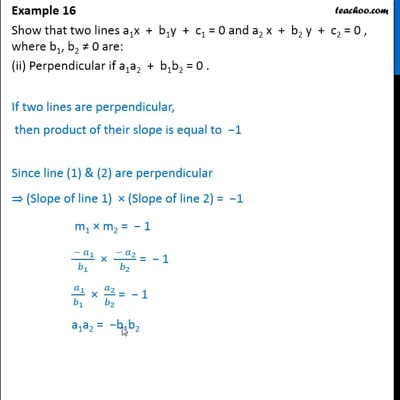This video is only available for Teachoo black users

Solve all your doubts with Teachoo Black (new monthly pack available now!)

### Transcript

Example 16 Show that two lines a1x + b1y + c1 = 0 and a2 x + b2 y + c2 = 0 , where b1, b2 ≠ 0 are: (i) Parallel if 𝑎_1/𝑏_1 = 𝑎2/𝑏2 The given lines are a1x + b1y + c1 = 0 & a2 x + b2 y + c2 = 0 Let slope of line (1) be m1 & slope of line (2) be m2 If two lines are parallel, then their slopes are equal If line (1) & (2) are parallel , then m1 = m2 Finding m1 & m2 From (1) a1x + b1y + c1 = 0 b1y = −c1 − a1 x b1y = −a1 x −c1 y = ( −𝑎_1 𝑥 − 𝑐_1)/𝑏_1 y = ((−𝑎_1)/𝑏_1 ) x –(𝑐_1/𝑏_1 ) The above equation is of the form y = mx + c where m is the slope Thus, Slope of line (1) = m1 = (−𝑎_1)/𝑏_1 From (2) a2x + b2y + c2 = 0 b2y = −c2 − a2 x b2y = −a2 x −c2 y = ( −𝑎_2 𝑥 − 𝑐_2)/𝑏_2 y = ((−𝑎_2)/𝑏_2 )x + (𝑐_2/𝑏_2 ) The above equation is of the form y = mx + c where m is the slope Thus, Slope of line (2) = m2 = (−𝑎_2)/𝑏_2 Since line (1) & (2) are parallel. So, m1 = m2 (−𝑎_1)/𝑏_1 = (−𝑎_2)/𝑏_2 ( 𝒂_𝟏)/𝒃_𝟏 = 𝒂_𝟐/𝒃_𝟐 Hence proved Example 16 Show that two lines a1x + b1y + c1 = 0 and a2 x + b2 y + c2 = 0 , where b1, b2 ≠ 0 are: (ii) Perpendicular if a1a2 + b1b2 = 0 . If two lines are perpendicular, then product of their slope is equal to −1 Since line (1) & (2) are perpendicular ⇒ (Slope of line 1) × (Slope of line 2) = −1 m1 × m2 = − 1 ( −𝑎_1)/𝑏_1 × ( −𝑎_2)/𝑏_2 = −1 ( 𝑎_1)/𝑏_1 × 𝑎_2/𝑏_2 = −1 a1a2 = −b1b2 a1a2 + b1b2 = 0 Hence proved